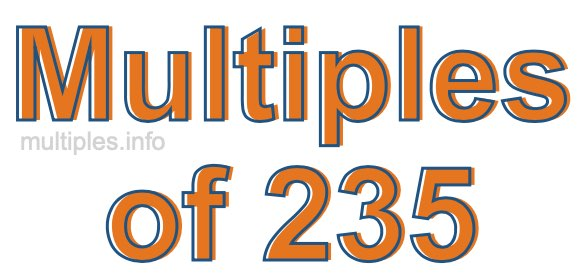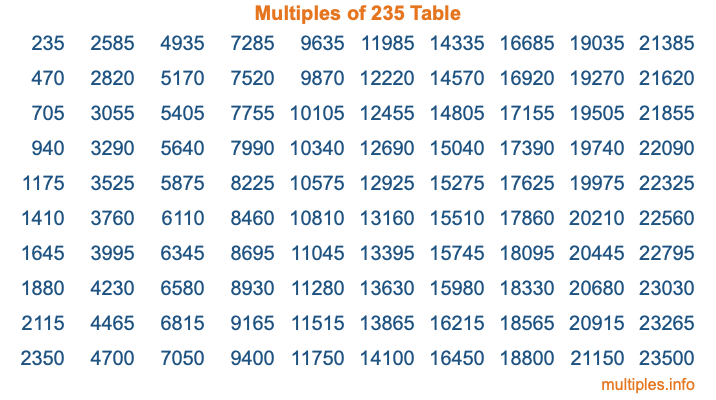Multiples of 235Welcome to the Multiples of 235 page. Here we will first teach you everything you will ever need to know about the multiples of 235, and then give you a study guide summary of everything we taught you to make sure you remember it all. Use this page to look up facts and learn information about the multiples of 235. This page will make you a multiples of two hundred thirty-five expert!

Definition of Multiples of 235
Multiples of 235 are all the numbers that when divided by 235 equal an integer. Each of the multiples of 235 are called a multiple. A multiple of 235 is created by multiplying 235 by an integer.

Therefore, to create a list of multiples of 235, you start with 1 multiplied by 235, then 2 multiplied by 235, then 3 multiplied by 235, and so on for as long as you want. Thus, the list of the first five multiples of 235 is 235, 470, 705, 940, and 1175. To see a larger list of multiples of 235, see the printable image of Multiples of 235 further down on this page. We also have a category where you can choose any nth multiple of 235.

Multiples of 235 Checker
The Multiples of 235 Checker below checks to see if any number of your choice is a multiple of 235. In other words, it checks to see if there is any number (integer) that when multiplied by 235 will equal your number. To do that, we divide your number by 235. If the the quotient is an integer, then your number is a multiple of 235.

Is  a multiple of 235?

Least Common Multiple of 235 and ...
A Least Common Multiple (LCM) is the lowest multiple that two or more numbers have in common. This is also called the smallest common multiple or lowest common multiple and is useful to know when you are adding our subtracting fractions. Enter one or more numbers below (235 is already entered) to find the LCM.

Check out our LCM Calculator if you need more details about the Least Common Multiple or if you need the LCM for different numbers for adding and subtraction fractions.

nth Multiple of 235
As we stated above, 235 is the first multiple of 235, 470 is the second multiple of 235, 705 is the third multiple of 235, and so on. Enter a number below to find the nth multiple of 235.

th multiple of 235

Multiples of 235 vs Factors of 235
235 is a multiple of 235 and a factor of 235, but that is where the similarities end. All postive multiples of 235 are 235 or greater than 235. All positive factors of 235 are 235 or less than 235.

Below is the beginning list of multiples of 235 and the factors of 235 so you can compare:

Multiples of 235: 235, 470, 705, 940, 1175, etc.

Factors of 235: 1, 5, 47, 235

As you can see, the multiples of 235 are all the numbers that you can divide by 235 to get a whole number. The factors of 235, on the other hand, are all the whole numbers that you can multiply by another whole number to get 235.

It's also interesting to note that if a number (x) is a factor of 235, then 235 will also be a multiple of that number (x).

Multiples of 235 vs Divisors of 235
The divisors of 235 are all the integers that 235 can be divided by evenly. Below is a list of the divisors of 235.

Divisors of 235: 1, 5, 47, 235

The interesting thing to note here is that if you take any multiple of 235 and divide it by a divisor of 235, you will see that the quotient is an integer.

Multiples of 235 Table
Below is an image of the first 100 multiples of 235 in a table. The table is in chronological order, column by column. The first column has the first ten multiples of 235, the second column has the next ten multiples of 235, and so on.The Multiples of 235 Table is also referred to as the 235 Times Table or Times Table of 235. You are welcome to print out our table for your studies.

Negative Multiples of 235
Although not often discussed or needed in math, it is worth mentioning that you can make a list of negative multiples of 235 by multiplying 235 by -1, then by -2, then by -3, and so on, to get the following list of negative multiples of 235:

-235, -470, -705, -940, -1175, etc.

Multiples of 235 Summary
Below is a summary of important Multiples of 235 facts that we have discussed on this page. To retain the knowledge on this page, we recommend that you read through the summary and explain to yourself or a study partner why they hold true.

There are an infinite number of multiples of 235.

A multiple of 235 divided by 235 will equal a whole number.

235 divided by a factor of 235 equals a divisor of 235.

The nth multiple of 235 is n times 235.

The largest factor of 235 is equal to the first positive multiple of 235.

235 is a multiple of every factor of 235.

235 is a multiple of 235.

A multiple of 235 divided by a divisor of 235 equals an integer.

235 divided by a divisor of 235 equals a factor of 235.

Any integer times 235 will equal a multiple of 235.

Multiples of a Number
Here you can get the multiples of another number, all with the same attention to detail as we did for multiples of 235 on this page.

Multiples of
Multiples of 236
Did you find our page about multiples of two hundred thirty-five educational? Do you want more knowledge? Check out the multiples of the next number on our list!

Copyright  |   Privacy Policy  |   Disclaimer  |   Contact